# HSSlive: Plus One & Plus Two Notes & Solutions for Kerala State Board

## BSEB Class 9 Science Chapter 12 Sound Textbook Solutions PDF: Download Bihar Board STD 9th Science Chapter 12 Sound Book AnswersBSEB Class 9 Science Chapter 12 Sound Textbook Solutions PDF: Download Bihar Board STD 9th Science Chapter 12 Sound Book Answers

BSEB Class 9th Science Chapter 12 Sound Textbooks Solutions and answers for students are now available in pdf format. Bihar Board Class 9th Science Chapter 12 Sound Book answers and solutions are one of the most important study materials for any student. The Bihar Board Class 9th Science Chapter 12 Sound books are published by the Bihar Board Publishers. These Bihar Board Class 9th Science Chapter 12 Sound textbooks are prepared by a group of expert faculty members. Students can download these BSEB STD 9th Science Chapter 12 Sound book solutions pdf online from this page.

## Bihar Board Class 9th Science Chapter 12 Sound Books Solutions

 Board BSEB Materials Textbook Solutions/Guide Format DOC/PDF Class 9th Subject Science Chapter 12 Sound Chapters All Provider Hsslive

## How to download Bihar Board Class 9th Science Chapter 12 Sound Textbook Solutions Answers PDF Online?

2. Click on the Bihar Board Class 9th Science Chapter 12 Sound Answers.
3. Look for your Bihar Board STD 9th Science Chapter 12 Sound Textbooks PDF.
4. Now download or read the Bihar Board Class 9th Science Chapter 12 Sound Textbook Solutions for PDF Free.

Find below the list of all BSEB Class 9th Science Chapter 12 Sound Textbook Solutions for PDF’s for you to download and prepare for the upcoming exams:

### Bihar Board Class 9 Science Chapter 12 Sound InText Questions and Answers

Page 162

Question 1.
How does the sound produced by a vibrating object in a medium reach your ear?
Sound after it is produced moves through a medium from the point of generation to the listener. When an object vibrates it sets the particle of the medium around it to vibrate. The particles do not travel all the way from the vibrating object to the ear. A particle of the medium in contact with the vibrating object is first displaced from its equilibrium position.

It then exerts a force on the adjacent particle as a result of which the adjacent particle gets displaced from its position of rest. After displacing the adjacent particle the first particle comes back to its original position. This process continues in the medium till the sound becomes feeble. The disturbance set by sound in the medium travels through the medium and not the particle of the medium.

Page 163

Question 1.
Explain how sound is produced by your school bell.
If it is an electric bell when the switch is pressed electric charges flow through the wire and the hammer strikes the gong. This produces vibrations. If it is a manual bell then vibrations are produced by hitting the round plate with a stick. When an object vibrates, then the air layers around it also start vibrating in exactly the same way. These layers of air carry sound waves from the sound producing object (bell in this case) to our ears. During the transmission no actual movement of air from the bell to our ear takes place. The air layers only vibrate back and forth and transfer the sound energy from one layer to the next layer till it reaches our ear.

Question 2.
Why are sound waves called mechanical waves?
A wave is a disturbance that moves through a medium and the particles of the medium set neighboui’ing particles into motion. They in turn produce similar motion in others and no particle moves away from its initial position. This exactly happens during propagation of sound in a medium, hence sound can be visualized as a wave. Sound waves are characterized by physical motion of particles in the medium and are called mechanical waves.

Question 3.
Suppose you and your friend are on the moon. Will you be able to hear any sound produced by your friend?
We cannot hear the sound produced by our friend because there is no atmosphere in the moon. It is all vacuum on the surface of moon. Sound needs a medium to travel.

Page 166

Question 1.
Which wave property determines
(a) loudness
(b) pitch?
(a) The loudness or softness of a sound is determined by its amplitude.
(b) The pitch of a sound is determined by its frequency.

Question 2.
Guess which sound has a higher pitch: guitar or car horn? .
Car horn has a higher pitch.

Page 166

Question 1.
What are wavelength, frequency, time period and amplitude of a sound wave?
(a) The distance between two consecutive crests or two consecutive troughs is called wavelength.
(b) The number of oscillations per unit time is called the frequency of the sound wave.
(c) The time period of a wave is the time taken by a wave to travel through a distance equal to its wavelength.
(d) The magnitude of the maximum displacement of the vibrating particles of the medium on either side of their mean undisturbed position is called the amplitude of the wave.
Or
The distance between two nearest points in a wave which are in the same phase of vibration is called the wavelength. It is represented by λ, (lambda). Its SI unit is metre (m).
(ii) The magnitude of the maximum displacement of the vibrating particles of the medium on either side of their mean undisturbed position is called the amplitude of the wave. The extent to which the medium is compressed when the sound wave passes through the medium measures the amplitude. It is usually represented by the letter ‘A’. Its SI unit is metre (m).

Question 2.
How are the wavelength and frequency of a sound wave related to its speed?
From the definition,
No. of waves produced in T s = 1
Then, No. of waves produced in 1 s = 1𝑇
Therefore, frequency, (v) = 1𝑇 s-1
We also know, that
Distance travelled by pne complete wave = λ
Time taken to complete one wave = T s
So, Distance travelled in 1 s = λ𝑇
But, distance travelled in 1 s is speed (υ).
υ = λ𝑇 = λυ (∴ 1𝑇 = v)
So, υ = λυ
Wave speed = Wavelength x Frequency The equation, υ = λυ, is called as the wave equation. The speed of sound remains same for all frequencies for a given medium under the same physical conditions.

Question 3.
Calculate thh wavelength of a sound wave whose frequency is 220 Hz and speed is 440 m/s in a given medium.
Speed, V.= 440 ms
Wavelength, λ = ?
Speed of the wave = Wavelength – Frequency
or V = λ x v
or 440 ms-1 = λ x 220 Hz
or λ = 1𝑇
The wavelength of the sound wave is 2 m.

Question 4.
A person is listening to a tone of 500 Hz sitting at a distance of 450 m from the source of the sound. What is the time interval between successive compressions from the source.
Frequency, v = 500 Hz
Time period, T = ?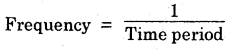v = 1𝑇
500 Hz = 1𝑇
T = 1500
T = 0.002s.
Thus, the time interval between successive compression from the source is 0.002 s.

Page 166

Question 1.
Distinguish between loudness and intensity of sound.
The amount of sound energy passing each i second through unit area is called intensity of sound. Loudness is a measure of the response of the ear to sound. Even though two sounds are of equal intensity, we can hear one as louder than the other because our ear can detect it better.

Page 167

Question 1.
In which of the three media, air, water or iron, does sound travel the fastest at a particular , temperature ?
Sound travels fastest in iron.

Page 168

Question 1.
An echo returned in 3 s. What is the distance of the reflecting surface from the source, given that the speed of sound is 342 m s-1?
Speed of sound, V = 342 ms-1
Time taken for hearing the echo, t = 3 s
Distance travelled by the sound
= V x t
= 342 ms-1 x 3 s = 1026 m
In 3 s sound Kas to travel twice the distance between the reflecting surface and the person. Hence the distance between the reflecting surface and the person
= 10262 = 513 m.

Page 169

Question 1.
Why are thq ceilings of concert hails curved?
Generally the ceilings of the concert halls, conference halls and cinema halls are curved so that sound after reflection reaches all corners of the hall as shown in Fig. 12.28. Sometimes a curved sound board may be placed behind the stage so that sound after reflecting at the sound board spreads evenly across the width of the hall. Sound waves having frequencies above 20,000 Hz and below 20 Hz cannot be heard by the normal human ear.

Page 169

Question 1.
Why are the ceilings of concert halls curved?
Generally the ceilings of the concert halls, conference halls and cinema halls are curved so that sound after reflection reaches all corners of the hail as shown in Fig. 12.28. Sometimes a curved sound board may be placed behind the stage so that sound after reflecting at the sound board spreads evenly across the width of the hail.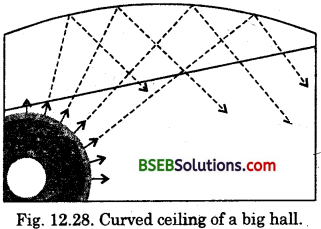Page 170

Question 1.
What is the audible range of the average human ear?
(i) Human ear is sensitive to sound intensity ranging from 20 – 180 dB (decibel).
(ii) The human ear is sensitive to only those vibrations whose frequency lies between 20 hertz (20 Hz) to 20b000 hertz (or 20 kilohertz, 20 kHz). This frequency range of hearing, from 20 Hz – 20 kHz, is called au’lible range.
Sound waves having frequencies above 20,000 Hz and below 20Hz cannot be heard by the normal human ear.

Question 2.
What is the range of frequencies associated with :
(а) Infrasound?
(b) Ultrasound?
(a) Infrasound : Sounds of frequencies below 20 Hz.
(b) Ultrasound : Sound of frequencies above 20 kHz.

Page 172

Question 1.
A submarine emits a sonar pulse, which returns from an underwater cliff in 1.02 s. If the speed of sound in soft water is 1531 ms-1, how far away is the cliff?
Time between transmission and detection, t – 1.02 s
Speed of ultrasound in sea water (salt water), V = 1531 ms2
Distance travelled by the sonar pulse = 2 x Depth of the sea = 2 x d
where d = depth of the sea.
Distance = Speed x Time
2d = V x t
2d = 1531 ms-1 x 1.02 s
2d = 1561.62 m
d = 1561.62𝑚2
d = 780.81
Thus, the distance of the cliff from the submarine is 780.81 m or 0.781 km.

### Bihar Board Class 9 Science Chapter 12 Sound Textbook Questions and Answers

Question 1.
What is sound and how is it produced?
(i) Sound is a form’of energy which usually produces the sensation of hearing in ears.
(ii) Sound can be produced in different ways. They are
(a) By stricking : For example, if we hit a metal plate with a stainless steel spoon and touch the plate gently we can feel the vibrations and also hear the sound.
(b) By plucking : When we pluck the wires of a guitar, sitar or any other string instrument the wires vibrate and a sound is produced.
(c) By blowing air : When we whistle through the mouth or play a flute the air column vibrates, so a sound is produced.
(d) By rubbing : When we rub our palm or drag a table on the floor a sound is produced.
Thus, we see that a sound is produced by a body only when it vibrates.

Question 2.
Describe with the help of a diagram how compressions and rarefactions are produced in air near a source of sound.
Air is the most common medium through which sound travels. A vibrating object when moves forward, first pushes the air in front of it and compress the air creating a region of high pressure. This region is called compression (G) as shown in Figure. It starts moving away from the surface of the vibrating object.

As this occurs the surface moves backward creating a region of low pressure called rarefaction (R) as shown in the figure. As the object vibrates i.e., moves back and forth rapidly, a series of’compression and rarefaction is set up in,the air. These make the sound to propagate through the medium.Question 3.
Cite an experiment to show that sound needs a material medium for its propagation.
Take an electric bell and an air tight glass bell jar. The electric bell is suspended inside the air tight bell jar connected to a vacuum pump as shown in figure on next page.
(i) When we press the switch we will be able to hear the bell.
(ii) When the air in the jar is pumped out gradually, the sound becomes fainter although the same current is passing through the hell.
(iii) When less air is left in the bell jar we will hear a very feeble sound.
(iv) When air is removed completely we will not be able to hear the sound of the bell.
(v) We can conclude that sound needs a medium to travel.Question 4.
Why is sound wave called a longitudinal wave?
When a sound wave travels, the air molecules oscillate in the same direction in which the sound waves propagate. Hence, sound waves are longitudinal waves.

Question 5.
Which characteristic of the sound helps you to identify your friend by his voice while sitting with others in a dark room.
The voice of different people have different frequency. How the brain interprets the frequency of an
emitted sound is called pitch. Hence the pitch of sound helps us to identify our friend by his voice while sitting with others in a dark room.

Question 6.
Flash and thunder are produced simultaneously. Bui thunder is heard a few seconds after the flash is seen. Why ?
Thunder is heard a few seconds after the flash is seen because the speed of light is more than the speed of sound.

Question 7.
A person has a hearing range from 20 Hz to 20 kHz. What are the typical wavelengths of sound waves in air corresponding to these two frequencies? Take the speed of sound in air as 344 ms-1.
Case I :
Frequency, v = 20 Hz
Speed of sound in air, V = 344 ms-1,
Wavelength, λ = ?
Speed = Wavelength x Frequency
V = λ x υ
344 ms-1 = λ x 20 Hz
λ = 344 ms−120 Hz = 17.2 m

Case II :
Frequency, v = 20 kHz = 20,000 Hz
Speed of sound in air,
V = 344 ms-1
Wavelength, λ = ?
Speed = Wavelength x Frequency
=> V = λ x v
344 ms-1 = λ x 20,000 Hz
λ = 344 ms−120,000 Hz = 0.0172

Thus, the wavelength of sound corresponding to 20 Hz and 20 kHz is 17.2 m and 0.172 m respectively.

Question 8.
Two children are at opposite ends of an aluminium rod. One strikes the end of the rod with a stone. Find the ratio of times taken by the sound wave in air and in aluminium to reach the second child.
Speed of sound in air,
V1 = 346 ms-1
Speed of sound in aluminium,
V2= 6420 ms-1.
Let the length of aluminium rod be x metres.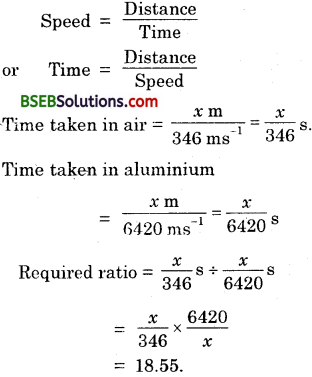Question 9.
The frequency of a source of sound is 10# Hz. How many times does it vibrate in a minute?
Frequency of sound = 100 Hz.
Number of vibrations in 1 minute (60 seconds) = 10 x 60 = 6000.

Question 10.
Does sound.follow the same laws of reflection as light does? Explain.
Yes, sound follows the same laws of reflection as light does.

The laws of reflection of sound are –
(i) The directions in which the sound is incident and reflected make equal angles with the normal to the reflecting surface.
(ii) The direction in which the sound is incident, the direction in which the sound is reflected and the normal to the reflecting surface all lie in the same plane.

Question 11.
When a sound is reflected from a distant object, an echo is produced. Let the distance between the reflecting surface and the source of sound production remains the same. Do you hear echo sound on a hotter day?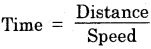i.e., Time is inversely proportional to the speed. In any medium, as we increase the temperature the speed of
sound increases. Thus on a hot day due to high temperature the speed of sound increases. Hence the time decreases. So we can hear the echo sooner.

Question 12.
Give two practical applications of reflection of sound waves.
(i) In stethoscopes used by the doctors, the sound of the patient’s heartbeat reaches the doctor’s ears by multiple reflection of sound.
(ii) Generally the ceilings of concert halls, conference halls and cinema halls are curved so that sound after reflection reaches all corners of the hall evenly.

Question 13.
A stone is dropped from the top of a tower 500 m high into a pond of water at the base of the tower! When is the splash heard at the top? Given, g = 10 ms-1 and speed of sound = 340 ms-1
Distance covered by stone (s) = 500 m
Initial velocity, u = 0 ms-1
Acceleration due to gravity,
g = 10 ms-2
S = ut + gt2
500 = 0 + 12 x 10 x t2
500 = 5 t2
t2 = 100
or t = 10s
Thus, in 10 s the stone reaches the pond.
Speed of sound = 340 ms-1
∴ Time taken by sound to cover
500 m = 500340 = 1.47 =1.5 s
∴ Total time taken = (10 + 1.5) s = 11.5 s
In 11.5 s the splash will be heard on top.

Question 14.
A sound wave travels at a speed of 339 m s-1. If its wavelength is 1.5 cm, what is the frequency of the wave? Will it be audible?
Speed = 339 ms-1
Wavelength = 1.5 cm 1.5340 = m = 0.015 m
Speed = Wavelength x Frequency
⇒ 339 ms-1 = 0.015 x Frequency
⇒ Frequency = 339 ms−10.015 m = 22600 Hz
It will not be audible to the human ear.

Question 15.
What is reverberation? How can it be reduced?
(i) A sound created in a big hall wall persist by repeated reflection from the walls until it is reduced to a value where it is no longer audible. The repeated reflection that results in this persistence of sound is called reverberation.
(ii) To reduce the undesirable effects due to reverberation, roof and walls of the auditorium are generally covered with sound absorbent materials like compressed fiborboard, rough plaster or draperies. The
seat materials are also selected having sound absorption properties. ,

Question 16.
What is loudness of sound? What factors does it depend on?
The loudness or softness of a sound depends upon its amplitude. The amplitude of the sound wave depends upon the force with which an object is made to vibrate. Loud sound can travel a larger distance as it is associated with higher energy whereas soft sound can travel a smaller distance as it, is associated with less energy. A sound wave spreads out after it leaves its source of production and as it moves away from the source its amplitude as well as its loudness decreases. Fig. 12.23 shows the wave shapes of a loud and soft sound of the same frequency. Loundness depends upon amplitude, intensity of sound and sensitivity of the ear.

Question 17.
Explain how bats use ultrasound to catch a prey.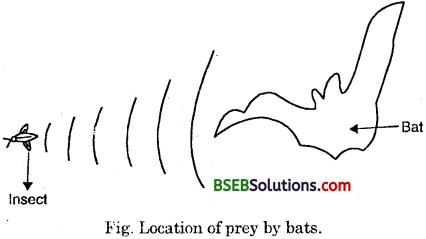Bats have weak eyes, so they cannot see their prey. They search out prey and fly in dark night by emitting and detecting reflections of ultrasonic waves. The high- pitched ultrasonic squeaks of the bat are reflected from the obstacles or prey and return to bat’s ear as shown in the figure. The nature of reflections tells the bat where the obstacle or prey is and what it is like.

Question 18.
How is ultrasound used for cleaning?
Ultrasound is used to clean parts located in hard to reach places i.e., spiral tube, odd shaped parts, electronic components etc. Objects to be cleaned are placed in a cleaning solution and ultrasonic waves are sent into the solution. Due to high frequency stir up or vibration, the particles of dust grease and dirt get detached and drop out. The objects get thoroughly cleaned.

Question 19.
Explain the working and application of a sonar.
SONAR employs ultrasonic waves for its working. Powerful pulses of ultrasound are sent out at regular intervals from a transmitter mounted on a ship. These pulses are intercepted by an object or the sea bottom, and get reflected. The reflected sound (or echo) is detected by an underwater receiver mounted on the ship known as detector. The time interval between transmission and reception of the ultrasonic signal is recorded. Knowing the speed of ultrasound in sea water and the time interval between transmission and reception of the ultrasound signal at a point, the depth of the sea bed or any other obstac.le at that point can be determined.

Let, Depth of the sea = d
Speed of sound in sea water = υ
Time lapse between transmission and reception of signal = t
So,
Time taken by the sound to travel distance, d = 𝑡2
Then,
Depth of the sea, d = υ x 𝑡2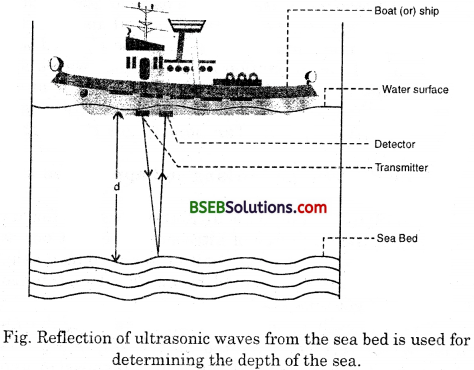Fig. Reflection of ultrasonic waves from the sea bed is used for determining the depth of the sea.

Question 20.
A sonar device on a submarine sends out a signal and receives an eacho 5 s later. Calculate the speed of sound in water if the distance of the object from the submarine is 3625 m.
Time between transmission and detection = 5 s
Distance of the object from the submarine = 3625 m
Distance travelled bv ultrasound = 2 x 3625 = 7250 m
Speed of sound in waterQuestion 21.
Explain how defects in a metal block can be detected by using ultrasound.
Metallic components are generally used in construction of big structures like buildings, bridges, machines and scientific equipments. The cracks or holes inside the metal blocks which are invisible from outside * reduces the strength of the structure. Ultrasonic waves are allowed to pass through the metal block and detectors are used to detect the transmitted waves. If there is a defect the ultrasound gets reflected back indicating the presence of flaws or defects.

Question 22.
Explain how the human ear works.
The outer ear is called ‘Pinna’. It collects the sound from the surrounding. The collected sound passes through the auditory canal. At the end of the auditory canal there is a thin membrane called the ear drum or tympanic membrane. When compression of the medium produced due to vibration of the object reaches the ear drums the pressure on the outside of the membrane increases and forces the eardrum inward. Similarly, the eardrum moves outward when a rarefaction reaches.

In this way the eardrum vibrates. The vibrations are amplified several times by three bones (the hammer, anvil and stirrup) in the middle ear which act as levers. The middle ear transmits the amplified pressure variations received from the sound wave to the inner ear. In the inner ear, the pressure variations are turned into electrical signals by the cochlea. These electrical signals are sent to the brain via the auditory nerve, and the brain interprets them as sound.

### Bihar Board Class 9 Science Chapter 12 Sound Textbook Activities

Activity 12.1a

Question 1.
Take a tuning fork and set it to vibration by striking its prong on a rubber pad.
(i) What will you find if you bring the vibrating tuning fork near your ear?
(ii) What will you feel if you touch your ear with one of the prongs of the vibrating tuning fork?
(i) If we bring the vibrating tuning fork near our ear we will be able to hear a sound.
(ii) If we touch our ear with one of the prongs of the vibrating tuning fork we can feel the vibration. ‘

Activity 12.1b

Suspend a table tennis ball or a small plastie ball by a thread from a support. You can suspend the ball using thread and a needle. Take a big needle and a thread, put a knot at one end of the thread. Then with tshe help of needle pass the thread through the ball.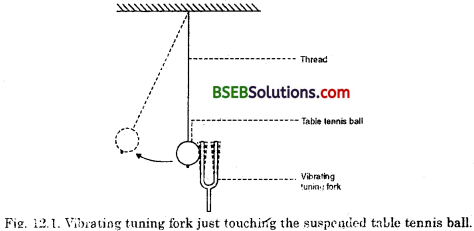(i) What will happen if you touch the ball with prong of the tuning fork without setting it into vibration?
(ii) What will happen if you touch the ball with a vibrating tuning fork?
(i) If we touch the ball with prong of the tuning fork without setting it into vibration there will be no change in the ball.
(ii) If we touch the ball with a vibrating tuning fork a sound will be produced due to the vibration of the tuning fork. This vibration will also set the ball into motion.

Activity 12.2

Question 1.
Fill water in a beaker or a glass up to its brim. Gently touch the water surface with one of the prongs of the vibrating tuning fork as shown in Fig. 12.2. Now dip the prongs of vibrating tuning fork in water as shown in Fig. 12.3.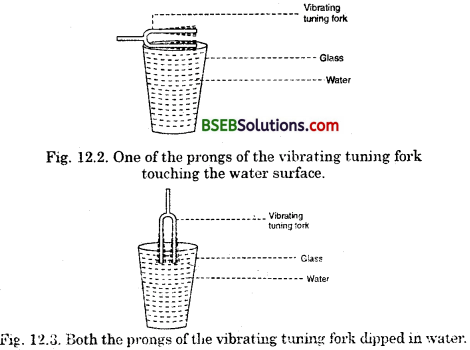(i) Observe what happens in both the cases?
(ii) Discuss with your friends why this happens.
(iii) What can you conclude from activity 12.1 and 12.2?
(i) In the first case the vibrating tuning fork will produce ripples in water which will move up and down. In the second case the vibrating tuning fork will produce ripples in water which will move sideways.
(ii) Sound is produced by a vibrating tuning fork which produces ripples in water.
(iii) We can conclude that sound cannot be produced without a vibrating body.

Activity 12.3
Question 1.
Make a list of different types of musical instruments and discuss with your friends which part of the instrument vibrates to produce sound.
Depending on the type of vibration the musical instruments are categorised into three main types. They are :

(a) Wind or reed instruments:
Different examples of wind instruments are shehnai, flute, nadaswaram, bugle, trumpet etc. In these instruments the air column vibrates and produces sound. The vibration is produced by blowing in; air through the mouth directly or through the reeds.

(b) Stringed instruments:
Different examples of stringed instruments are guitar, sitar, veena, tanpura, violin etc. The stretched strings of these instruments vibrate when they are plucked or played with a bow. The pitch of the sound produced by these instruments can be altered by changing the length of the strings.

(c) Percussion instruments:
Different examples of percussion instruments are tabla, mridangam, drum, tambourine (dafli) etc. In these instruments vibration is produced by a stretched membrane when stuck by hand or a drum stick. There is a fourth category of instrument which is used mainly in India. It is called Ghana Vadya. Different examples of ghana vadya are ghatam, noot (mudpots), manjira, jal tarang etc. Vibration is produced in these instruments by stricking them in rhythmic manner.

Activity 12.4

• Take a slinky. Ask your friend to hold one end. You hold the other end. Now stretch the slinky as shown in Fig. 12.8. (a). Then give it a sharp push towards your friend.
• What do you notice? If you move your hand pushing and pulling the slinky alternatively, what will you observe?
• If you mark a dot on the slinky, you will observe that the dot on the slinky will move back and forth parallel to the direction of

the propagation of the disturbance.Fig. 12.8. Longitudinal wave in a slinky.
(i) When we give a small jerk a small hump will be produced. This hump in the slinky is called a pulse. This hump travels forward slowly.
If we give a sharp push a continuous disturbance will be produced in the slinky.
(ii) If we move our hand pushing and pulling the slinky alternatively, the slinky will move back and forth parallel to the direction of the propagation of the disturbance. This can be observed clearly by marking a dot on the slinky.

Activity 12.5

Question 1.
Take two identical pipes, as shown in Fig. 12.23. You can make the pipes using chart paper. The length of the pipes should be sufficiently long as shown paper. Arrange them on a table near a wall. Keep a clock near the open end of one of the pipes, say pipe A and try to hear the sound of the clock through the other pipe. Put a plywood sheet . between the two tubes perpendicular to the wall. This does not allow the direct hearing of the ticking 1 sound of the clock. Adjust the position of the pipes so that you can best hear the sound of the clock.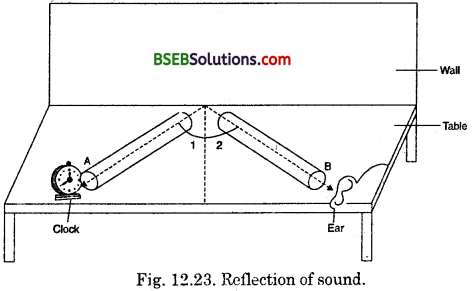(i) Now measure the angles of incidence and reflection and see the relationship between the angles.
(ii) Lift the pipe on the right vertically to a small height and observe what happens?
(i) We will find that angle of incidence (1) and angle of reflection (2) are equal.
(ii) If we lift the pipe on the right vertically to a small height we will not be able to hear the ticking sound of the clock as the reflected sound will not travel through pipe B.

### Bihar Board Class 9 Science Chapter 12 Sound Additional Important Questions and answers

Question 1.
Name few sources of sound which we hear everyday.
Various sources of sounds are human beings, birds, animals, bells, machines, vehicles, various electrical gadgets etc.

Question 2.
What is sound?
Sound is a form of energy which usually produces the sensation of hearing in ears.

Question 3.
Name some other forms of energy.
Mechanical energy, heat energy, light energy etc.

Question 4.
What happens when you clap your hands?
Sound is produced when we clap our hands.

Question 1.
(i) What is wave motion ?
(ii) Does a wave require a material medium for its propagation?
(i.) Wave motion is the. periodic disturbance produced in a medium by a vibrating (oscillating) body.
(ii) Yes, a wave requires material medium for its propagation.

Question 2.
What are the characteristics of wave motion?
(i) During wave motion, the particles of the medium vibrate about their mean position. The particles of the medium do not travel from one place to another.
(ii) Wave motion travels at the same speed in all directions.
(iii) During wave motion, energy is transferred from one point to another. There is no transfer of matter through the medium.
(iv) During wave motion, the medium does not move as a whole. Only the disturbance travels through the medium.

Question 3.
What characteristics should the medium possess for a wave to travel through it?
For a wave to travel through a medium, the medium
(i) should possess inertia or mass so that kinetic energy can be stored.
(ii) be elastic, so that potential energy can be stored.
(iii) should have uniform density.
(iv) should have minimum frictional force between the particles, so that the loss of energy is minimum.

Question 1.
How is the depth of sea or the location of a submerged object determined by SONAR method?
Or
What is echo-ranging?
SONAR employs ultrasonic waves for its working. Powerful pulses of ultrasound are sent out at regular intervals from a transmitter mounted on a ship. These pulses are intercepted by an object or the sea bottom, and get reflected. The reflected sound (or echo) is detected by an underwater receiver mounted on the ship known a? detector. The time interval between transmission and reception of the ultrasonic signal is recorded. Knowing the speed of ultrasound in sea water and the time interval between transmission and reception of the ultrasound signal at a point, the depth of the sea bed or any other obstacle at that point can be determined.
Let,
Depth of the sea = d
Speed of sound in sea water = υ
Time lapse between transmission and reception of signal = t
So,
Time taken by the sound to travel distance, d = 𝑡2
Then,
.Depth of the sea, d = υ x 𝑡2

## Bihar Board Class 9th Science Chapter 12 Sound Textbooks for Exam Preparations

Bihar Board Class 9th Science Chapter 12 Sound Textbook Solutions can be of great help in your Bihar Board Class 9th Science Chapter 12 Sound exam preparation. The BSEB STD 9th Science Chapter 12 Sound Textbooks study material, used with the English medium textbooks, can help you complete the entire Class 9th Science Chapter 12 Sound Books State Board syllabus with maximum efficiency.

## FAQs Regarding Bihar Board Class 9th Science Chapter 12 Sound Textbook Solutions

#### Can we get a Bihar Board Book PDF for all Classes?

Yes you can get Bihar Board Text Book PDF for all classes using the links provided in the above article.

## Important Terms

Bihar Board Class 9th Science Chapter 12 Sound, BSEB Class 9th Science Chapter 12 Sound Textbooks, Bihar Board Class 9th Science Chapter 12 Sound, Bihar Board Class 9th Science Chapter 12 Sound Textbook solutions, BSEB Class 9th Science Chapter 12 Sound Textbooks Solutions, Bihar Board STD 9th Science Chapter 12 Sound, BSEB STD 9th Science Chapter 12 Sound Textbooks, Bihar Board STD 9th Science Chapter 12 Sound, Bihar Board STD 9th Science Chapter 12 Sound Textbook solutions, BSEB STD 9th Science Chapter 12 Sound Textbooks Solutions,
Share: